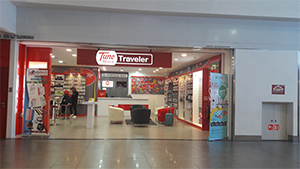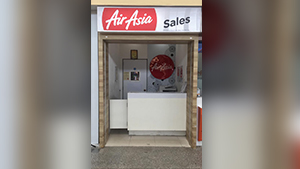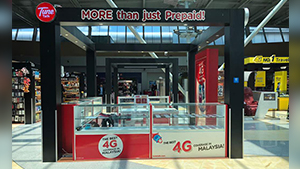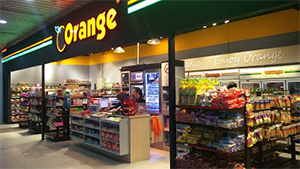## TT KIOSK LOCATION#### Tune Store Traveler (KLIA2) See Store Location

Lot No L2-73A Level 2, Gateway@KLIA2, Terminal KLIA2, KL International Airport, Jalan KLIA2/1, 64000 Sepang, Selangor.#### Langkawi Airport (AirAsia ATSC) See Store Location

AIRASIA SALES LANGKAWI, Ground Floor Langkawi International Airport, Mukim Padang Matsirat, 07100 Langkawi, Kedah.#### Johor Baharu Airport See Store Location

Lot KA6, Aeromall, Senai International Airport, 81250, Johor Baharu, Johor.#### Kota Kinabalu Airport See Store Location

Orange Convenient Shop, Unit L1L01, Terminal 1, Level 1,Public Concourse, Kota Kinabalu International Airport, 88740. Sabah.#### Penang Airport See Store Location

L1LFB03A, WL1, Arrival Hall, Penang International Airport, 11900, Bayan Lepas, Pulau Pinang.
(Tune Talk Store is located inside Communication Center in Level 1)

Response time: 1.2082939147949
1. select * from `rainlab_translate_attributes` where `locale` = ? and `model_id` = ? and `model_type` = ? limit 1
2. select * from `rainlab_translate_attributes` where `locale` = ? and `model_id` = ? and `model_type` = ? limit 1
3. select * from `rainlab_translate_attributes` where `locale` = ? and `model_id` = ? and `model_type` = ? limit 1
4. select * from `rainlab_translate_attributes` where `locale` = ? and `model_id` = ? and `model_type` = ? limit 1
5. select * from `rainlab_translate_attributes` where `locale` = ? and `model_id` = ? and `model_type` = ? limit 1
6. select * from `rainlab_translate_attributes` where `locale` = ? and `model_id` = ? and `model_type` = ? limit 1
7. select * from `rainlab_translate_attributes` where `locale` = ? and `model_id` = ? and `model_type` = ? limit 1
8. select * from `rainlab_translate_attributes` where `locale` = ? and `model_id` = ? and `model_type` = ? limit 1
9. select * from `rainlab_translate_attributes` where `locale` = ? and `model_id` = ? and `model_type` = ? limit 1
10. select * from `rainlab_translate_attributes` where `locale` = ? and `model_id` = ? and `model_type` = ? limit 1
11. select * from `rainlab_translate_attributes` where `locale` = ? and `model_id` = ? and `model_type` = ? limit 1
12. select * from `rainlab_translate_attributes` where `locale` = ? and `model_id` = ? and `model_type` = ? limit 1
13. select * from `rainlab_translate_attributes` where `locale` = ? and `model_id` = ? and `model_type` = ? limit 1
14. select * from `rainlab_translate_attributes` where `locale` = ? and `model_id` = ? and `model_type` = ? limit 1
15. select * from `rainlab_translate_attributes` where `locale` = ? and `model_id` = ? and `model_type` = ? limit 1
16. select * from `rainlab_translate_attributes` where `locale` = ? and `model_id` = ? and `model_type` = ? limit 1
17. select * from `rainlab_translate_attributes` where `locale` = ? and `model_id` = ? and `model_type` = ? limit 1
18. select * from `rainlab_translate_attributes` where `locale` = ? and `model_id` = ? and `model_type` = ? limit 1
19. select * from `rainlab_translate_attributes` where `locale` = ? and `model_id` = ? and `model_type` = ? limit 1
20. select * from `rainlab_translate_attributes` where `locale` = ? and `model_id` = ? and `model_type` = ? limit 1
21. select * from `rainlab_translate_attributes` where `locale` = ? and `model_id` = ? and `model_type` = ? limit 1
22. select * from `rainlab_translate_attributes` where `locale` = ? and `model_id` = ? and `model_type` = ? limit 1
23. select * from `rainlab_translate_attributes` where `locale` = ? and `model_id` = ? and `model_type` = ? limit 1
24. select * from `rainlab_translate_attributes` where `locale` = ? and `model_id` = ? and `model_type` = ? limit 1
25. select * from `rainlab_translate_attributes` where `locale` = ? and `model_id` = ? and `model_type` = ? limit 1
26. select * from `rainlab_translate_attributes` where `locale` = ? and `model_id` = ? and `model_type` = ? limit 1
27. select * from `rainlab_translate_attributes` where `locale` = ? and `model_id` = ? and `model_type` = ? limit 1
28. select * from `rainlab_translate_attributes` where `locale` = ? and `model_id` = ? and `model_type` = ? limit 1
29. select * from `rainlab_translate_attributes` where `locale` = ? and `model_id` = ? and `model_type` = ? limit 1
30. select * from `rainlab_translate_attributes` where `locale` = ? and `model_id` = ? and `model_type` = ? limit 1
31. select * from `rainlab_translate_attributes` where `locale` = ? and `model_id` = ? and `model_type` = ? limit 1
32. select * from `rainlab_translate_attributes` where `locale` = ? and `model_id` = ? and `model_type` = ? limit 1
33. select * from `rainlab_translate_attributes` where `locale` = ? and `model_id` = ? and `model_type` = ? limit 1
34. select * from `rainlab_translate_attributes` where `locale` = ? and `model_id` = ? and `model_type` = ? limit 1
35. select * from `rainlab_translate_attributes` where `locale` = ? and `model_id` = ? and `model_type` = ? limit 1
36. select * from `rainlab_translate_attributes` where `locale` = ? and `model_id` = ? and `model_type` = ? limit 1
37. select * from `rainlab_translate_attributes` where `locale` = ? and `model_id` = ? and `model_type` = ? limit 1
38. select * from `rainlab_translate_attributes` where `locale` = ? and `model_id` = ? and `model_type` = ? limit 1
39. select * from `rainlab_translate_attributes` where `locale` = ? and `model_id` = ? and `model_type` = ? limit 1
40. select * from `rainlab_translate_attributes` where `locale` = ? and `model_id` = ? and `model_type` = ? limit 1
41. select * from `rainlab_translate_attributes` where `locale` = ? and `model_id` = ? and `model_type` = ? limit 1
42. select * from `rainlab_translate_attributes` where `locale` = ? and `model_id` = ? and `model_type` = ? limit 1
43. select * from `rainlab_translate_attributes` where `locale` = ? and `model_id` = ? and `model_type` = ? limit 1
44. select * from `rainlab_translate_attributes` where `locale` = ? and `model_id` = ? and `model_type` = ? limit 1
45. select * from `rainlab_translate_attributes` where `locale` = ? and `model_id` = ? and `model_type` = ? limit 1
46. select * from `rainlab_translate_attributes` where `locale` = ? and `model_id` = ? and `model_type` = ? limit 1
47. select * from `rainlab_translate_attributes` where `locale` = ? and `model_id` = ? and `model_type` = ? limit 1
48. select * from `rainlab_translate_attributes` where `locale` = ? and `model_id` = ? and `model_type` = ? limit 1
49. select * from `rainlab_translate_attributes` where `locale` = ? and `model_id` = ? and `model_type` = ? limit 1
50. select * from `rainlab_translate_attributes` where `locale` = ? and `model_id` = ? and `model_type` = ? limit 1
51. select * from `rainlab_translate_attributes` where `locale` = ? and `model_id` = ? and `model_type` = ? limit 1
52. select * from `rainlab_translate_attributes` where `locale` = ? and `model_id` = ? and `model_type` = ? limit 1
53. select * from `rainlab_translate_attributes` where `locale` = ? and `model_id` = ? and `model_type` = ? limit 1
54. select * from `rainlab_translate_attributes` where `locale` = ? and `model_id` = ? and `model_type` = ? limit 1
55. select * from `rainlab_translate_attributes` where `locale` = ? and `model_id` = ? and `model_type` = ? limit 1
56. select * from `rainlab_translate_attributes` where `locale` = ? and `model_id` = ? and `model_type` = ? limit 1
57. select * from `rainlab_translate_attributes` where `locale` = ? and `model_id` = ? and `model_type` = ? limit 1
58. select * from `rainlab_translate_attributes` where `locale` = ? and `model_id` = ? and `model_type` = ? limit 1
59. select * from `rainlab_translate_attributes` where `locale` = ? and `model_id` = ? and `model_type` = ? limit 1
60. select * from `rainlab_translate_attributes` where `locale` = ? and `model_id` = ? and `model_type` = ? limit 1
61. select * from `rainlab_translate_attributes` where `locale` = ? and `model_id` = ? and `model_type` = ? limit 1
62. select * from `rainlab_translate_attributes` where `locale` = ? and `model_id` = ? and `model_type` = ? limit 1
63. select * from `rainlab_translate_attributes` where `locale` = ? and `model_id` = ? and `model_type` = ? limit 1
64. select * from `rainlab_translate_attributes` where `locale` = ? and `model_id` = ? and `model_type` = ? limit 1
65. select * from `rainlab_translate_attributes` where `locale` = ? and `model_id` = ? and `model_type` = ? limit 1
66. select * from `rainlab_translate_attributes` where `locale` = ? and `model_id` = ? and `model_type` = ? limit 1
67. select * from `rainlab_translate_attributes` where `locale` = ? and `model_id` = ? and `model_type` = ? limit 1
68. select * from `rainlab_translate_attributes` where `locale` = ? and `model_id` = ? and `model_type` = ? limit 1
69. select * from `rainlab_translate_attributes` where `locale` = ? and `model_id` = ? and `model_type` = ? limit 1
70. select * from `rainlab_translate_attributes` where `locale` = ? and `model_id` = ? and `model_type` = ? limit 1
71. select * from `rainlab_translate_attributes` where `locale` = ? and `model_id` = ? and `model_type` = ? limit 1
72. select * from `rainlab_translate_locales` where `code` = ? limit 1
73. select * from `rainlab_translate_attributes` where `locale` = ? and `model_id` = ? and `model_type` = ? limit 1
74. select * from `rainlab_translate_attributes` where `locale` = ? and `model_id` = ? and `model_type` = ? limit 1